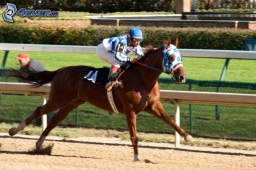# Combined 22681

The farm keeps cows, horses, and sheep. There are 37 cows, which is 25 more than the sheep. There are seven times fewer horses than cows and sheep combined. How many animals do they keep on the farm together?

x =  56

### Step-by-step explanation:Did you find an error or inaccuracy? Feel free to write us. Thank you!

Tips for related online calculators
Need help calculating sum, simplifying, or multiplying fractions? Try our fraction calculator.
Do you have a linear equation or system of equations and looking for its solution? Or do you have a quadratic equation?